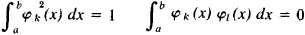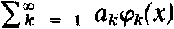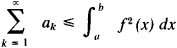Bessel Inequality

(redirected from Bessel's inequality)
Also found in: Wikipedia.

Bessel inequality

[′bes·əl ‚in·ē′kwäl·əd·ē]
(mathematics)
The statement that the sum of the squares of the inner product of a vector with the members of an orthonormal set is no larger than the square of the norm of the vector.
McGraw-Hill Dictionary of Scientific & Technical Terms, 6E, Copyright © 2003 by The McGraw-Hill Companies, Inc.
The following article is from The Great Soviet Encyclopedia (1979). It might be outdated or ideologically biased.

Bessel Inequality

an inequality for the coefficients of the Fourier series for an arbitrary orthonormalized system of functions φk (x) (k = 1, 2,. . .)—that is, a system defined over a certain interval [a, b] and satisfying the conditions (k ≠;l)If the function f(x) is measurable over the interval [a, b], and the function f2{x) is integrable over this interval, andis a Fourier series of f(x) for the system φκ ((x), then the Bessel inequalityis valid.

The Bessel inequality plays an important role in all investigations which pertain to the theory of orthogonal series. In particular, it shows that the Fourier coefficients of the function f(x) tend to zero as n → ∞ . For a trigonometric system of functions this inequality was obtained by F. Bessel (1828). If the system of functions φk is such that for any function f the Bessel inequality is converted into an equality, then it is called the Parseval equality.

S. B. STECHKIN